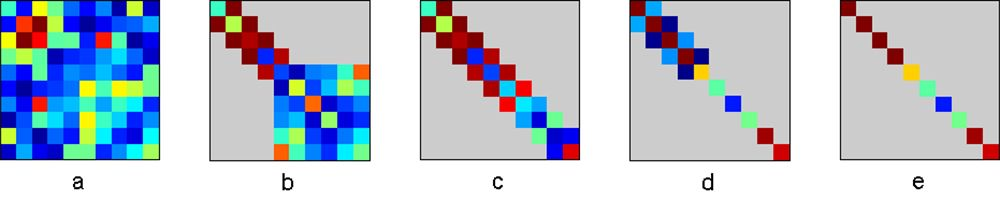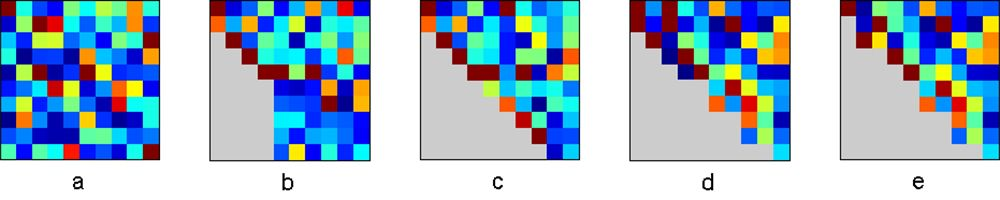# Variants of the QR Algorithm

By Cleve Moler, MathWorks

The QR algorithm is one of the most successful and powerful tools we have in mathematical software.

The MATLAB® core library includes several variants of the QR algorithm. These variants compute the eigenvalues of real symmetric matrices, real nonsymmetric matrices, pairs of real matrices, complex matrices, pairs of complex matrices, and singular values of various types of matrices. These core library functions are used in various MATLAB toolboxes to find eigenvalues and singular values of sparse matrices and linear operators, find zeros of polynomials, solve special linear systems, assess numerical stability, and perform many other tasks.

Dozens of people have contributed to the development of the QR algorithm variants. The first papers on the subject came from J.G.F. Francis in 1961 and 1962 and Vera N. Kublanovskaya in 1963. But it was J.H. Wilkinson who developed the first complete implementation of the QR algorithm. Wilkinson also developed an important convergence analysis. Wilkinson’s book The Algebraic Eigenvalue Problem and two of his papers were published in 1965. This means we’ll be able to celebrate 2015 as the golden anniversary of the practical QR algorithm.

The variant of the QR algorithm used for the singular value decomposition (SVD) was published in 1965 by Gene Golub and Velvel Kahan and perfected in 1969 by Golub and Christian Reinsch.

## The Name “QR”

The name “QR” is derived from the letter Q, used to denote orthogonal matrices, and the letter R, used to denote right triangular matrices. There is a qr function in MATLAB, but it computes the QR factorization, not the QR algorithm. Any matrix, whether real or complex, square or rectangular, can be factored into the product of a matrix Q with orthonormal columns and matrix R that is nonzero only in its upper, or right, triangle. You might remember the Gram Schmidt process, which does pretty much the same thing, although in its original form it is numerically unstable.

## A One-Liner

Using the qr function, a simple variant of the QR algorithm can be expressed in one line of MATLAB code. Let A be a square, n-by-n matrix, and let I = eye(n,n). Then one step of the QR iteration is given by

s = A(n,n); [Q,R] = qr(A - s*I);A = R*Q + s*I


The quantity $$s$$ is the shift; it accelerates convergence. As $$A$$ approaches an upper triangular matrix, $$s$$ approaches an eigenvalue. If you enter these three statements on a single line, you can use the up-arrow key to iterate.

The QR factorization produces an upper triangular $$R$$.

$A-sI = QR$

Then the reverse order multiplication, $$RQ$$, restores the eigenvalues because

$RQ + sl = Q^{T}(A - sl)Q + sl = Q^{T}AQ$

So the new $$A$$ is similar to the original $$A$$. Each iteration effectively transfers some “mass” from the lower to the upper triangle while preserving the eigenvalues. As the iterations proceed, the matrix begins to approach an upper triangular matrix with the eigenvalues conveniently displayed on the diagonal.

## An Example

To illustrate this process we’ll use a matrix from the MATLAB Gallery collection.

A = gallery(3)
A =
-149  -50  -154
537  180   546
-27   -9   -25


It is not at all obvious, but this matrix has been constructed to have eigenvalues 1, 2, and 3. The first iteration of our one-line QR code produces

 A =
28.8263  -259.8671  773.9292
1.0353    -8.6686   33.1759
-0.5973     5.5786  -14.1578


The matrix is now much nearer to being upper triangular, but the eigenvalues are still not evident. However, after five more iterations we have

A =
3.0321  -8.0851  804.6651
0.0017   0.9931  145.5046
-0.0001   0.0005    1.9749


We begin to see the eigenvalues 3, 1, and 2 emerging on the diagonal. Eight more iterations give

A =
3.0716  -7.6952  802.1201
0.0193   0.9284  158.9556
0        0    2.0000


The eigenvalue 2.0 has been computed to the displayed accuracy, and the below-diagonal element adjacent to it has become zero. At this point it is necessary to continue the iteration on the 2-by-2 upper left submatrix.

The QR algorithm is never carried out in this simple form. It is always preceded by a reduction to a compact form in which all the elements below the subdiagonal are zero. The iteration preserves this reduced form, and the factorizations can be done much more quickly. The shift strategy is more sophisticated, and is different for various forms of the algorithm. Additionally, the reduced form is of utmost importance for the convergence properties of the iteration.

## Symmetric Matrices

Figures 1–3 illustrate three of the most important variants of the QR algorithm. The figures are snapshots taken from the output generated by the program eigsvdgui.m from Numerical Computing with MATLAB.

The simplest variant involves real, symmetric matrices. An n-by-n real, symmetric matrix can be reduced to tridiagonal form by means of n-2 Householder reflections, which are a sequence of similarity transformations preserving the eigenvalues. The QR iteration applies to the tridiagonal form. Wilkinson provided a shift strategy that allowed him to prove both global convergence and a local cubic convergence rate. Even in the presence of roundoff error, this algorithm is guaranteed to succeed.

Figure 1 shows an initial symmetric matrix, the situation halfway through the reduction to tridiagonal, the tridiagonal, the situation partway through the QR iteration, and finally, the eigenvalues. Actually, because the matrix is symmetric, the computation is only performed on one half of the array, but our figure reflects the results to show an entire matrix.Figure 1. Eigenvalues of a symmetric matrix. Left to right: The input—a random symmetric 10-by-10 matrix, halfway through the orthogonal reduction to tridiagonal form, symmetric tridiagonal form, partway through the symmetric tridiagonal QR iteration, and the final diagonal matrix of eigenvalues.

## Nonsymmetric Matrices

The situation for real, nonsymmetric matrices is much more complicated. The initial reduction uses n-2 Householder similarity transformations to create a Hessenberg matrix, which is upper triangular plus an “extra” subdiagonal. A QR iteration with a double shift strategy is then used. This preserves the Hessenberg form while attempting to create a real Schur form, which is upper triangular except for 2-by-2 blocks corresponding to pairs of complex conjugate eigenvalues on the diagonal.

The nonsymmetric Hessenberg QR algorithm is not infallible. It is an iterative process that is not always guaranteed to converge. Even 30 years ago, counterexamples to the basic iteration were known. Wilkinson introduced an additional “ad hoc” shift to handle them, but no one has been able to prove a complete convergence theorem. So, on rare occasions, MATLAB users might see this message:

Error using ==> eig, Solution will not converge


Years ago, recipients of this message might have accepted it as unavoidable. But today, most people would be surprised or annoyed; they have come to expect infallibility.

We now know of a 4-by-4 example that may cause the real, nonsymmetric QR algorithm to fail on certain computers, even with Wilkinson’s ad hoc shift. The matrix is

$A = \begin{bmatrix} 0 & 2 & 0 & -1 \\ 1 & 0 & 0 & 0 \\ 0 & 1 & 0 & 0 \\ 0 & 0 & 1 & 0 \end{bmatrix}$

This is the companion matrix of the polynomial $$p(x)=x^4 - 2x^2 + 1$$, and the statement

roots([1 0 -2 0 1])


calls for the computation of eig(A). The values $$\lambda = 1$$ and $$\lambda = -1$$ are both eigenvalues, or polynomial roots, with multiplicity two. For real $$x$$, the polynomial $$p(x)$$ is never negative. These double roots slow down the iteration so much that, on some computers, the vagaries of roundoff error interfere before convergence is detected. The iteration can wander forever, trying to converge but veering off when it gets close.

Similar behavior is shown by examples of the form

$\begin{bmatrix}0 & 1 & 0 & 0 \\ 1 & 0 & -\delta & 0 \\ 0 & \delta & 0 & 1 \\ 0 & 0 & 1 & 0 \end{bmatrix}$

where $$\delta$$ is small but not small enough to be neglected—say, $$\delta = 10^{-8}$$. The exact eigenvalues are close to a pair of double roots. The Wilkinson double shift iteration uses one eigenvalue from each pair. This iteration does change the matrix, but not enough to get rapid convergence. So we have to use a different double shift based on repeating one of the eigenvalues of the lower 2-by-2 blocks.

These different ad hoc shift strategies have been incorporated into the latest versions of LAPACK, and consequently, into MATLAB. We are now in the situation where we do not know of any matrices that cause eig or roots to display the “will not converge” message, but we have no proof that the nonsymmetric code with these various embellishments is infallible.

Figure 2 shows an initial nonsymmetric matrix, the situation halfway through the reduction to Hessenberg form, the Hessenberg form, the situation partway through the QR iteration, and the final real Schur form. For this particular matrix, it happens there are four real eigenvalues and three complex conjugate pairs, for a total of ten eigenvalues.Figure 2. Eigenvalues of a nonsymmetric matrix. Left to right: The input—a random 10-by-10 matrix; halfway through the orthogonal reduction to Hessenberg form; Hessenberg form, upper triangular plus one subdiagonal; partway through the nonsymmetric QR iteration; and the final real Schur form with real eigenvalues and 2-by-2 blocks with complex pairs of eigenvalues on the diagonal.

## Singular Values

The singular values of a possibly rectangular matrix $$A$$ are the square roots of the eigenvalues of the symmetric matrix $$A^{T}A$$. This fact can be used to motivate and analyze an algorithm, but it should not be the basis for actual computation with finite precision arithmetic. The initial phase of the Golub-Kahan-Reinsch algorithm involves Householder reflections operating on both the left and the right to reduce a matrix to a bidiagonal form. This phase is followed by an SVD variant of the QR algorithm operating on the bidiagonal. Wilkinson’s analysis of symmetric tridiagonal QR applies to this algorithm as well, so the process is guaranteed to be globally convergent.

Figure 3 shows an initial rectangular matrix, the situation halfway through the reduction to bidiagonal form, the bidiagonal form, the situation partway through the QR iteration, and the final diagonal form containing the singular values.Figure 3. Singular values of a rectangular matrix. Left to right: The input—a random rectangular 12-by-10 matrix, halfway through the orthogonal reduction to bidiagonal form, bidiagonal form, partway through the bidiagonal singular value QR iteration, and the final diagonal matrix of singular values.

## QR Algorithm Applications

While the QR algorithms for computing eigenvalues and singular values are closely related, the applications of the results are usually very different. Eigenvalues are often employed to analyze systems of ordinary differential equations where behavior as a function of time is important. Singular values, on the other hand, are useful for analyzing static systems of simultaneous linear equations, where the number of equations is often not the same as the number of unknowns.

Control theory and control design automation make heavy use of eigenvalues. The classical state-space system of differential equations studied in control theory is

\begin{align}\dot{x} &= Ax + Bu \\ y &= Cx+Du \end{align}

Using the QR algorithm to compute the eigenvalues of $$A$$ is essential to the investigation of issues like stability and controllability.

In statistics, the SVD is a numerically reliable way to obtain the principal components. Principal component analysis (PCA) is a technique for analyzing an overdetermined system of simultaneous linear equations

$Ax=b$

where $$A$$ has more rows than columns. Using the QR algorithm to compute the singular values and vectors of $$A$$ produces the principal components.

## For Further Reading and Viewing

Golub, Gene H., and Charles F. Van Loan, Matrix Computations, 4th Edition, Johns Hopkins University Press, 1996, 697pp.

Moler, Cleve, Numerical Computing with MATLAB, Chapter 10, “Eigenvalues and Singular Values.”

Moler, Cleve, 1976 Matrix Singular Value Decomposition Film.

Wilkinson, J.H., The Algebraic Eigenvalue Problem, Oxford University Press, 1965, 662 pp.